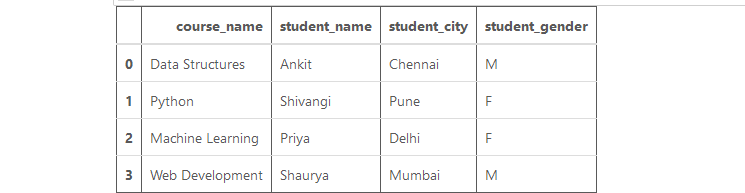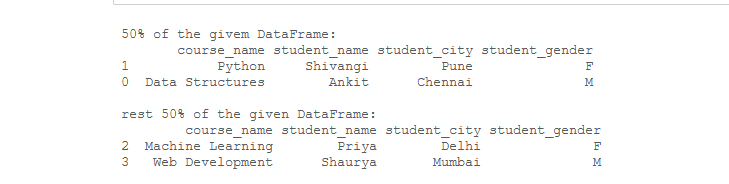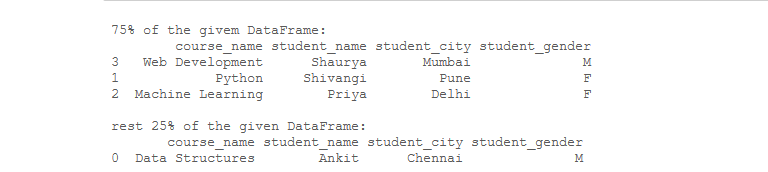# Divide a Pandas DataFrame randomly in a given ratio

• Last Updated : 25 Oct, 2021

Divide a Pandas Dataframe task is very useful in case of split a given dataset into train and test data for training and testing purposes in the field of Machine Learning, Artificial Intelligence, etc.  Let’s see how to divide the pandas dataframe randomly into given ratios. For this task, We will use Dataframe.sample() and Dataframe.drop() methods of pandas dataframe together.

The Syntax of these functions are as follows –

Attention geek! Strengthen your foundations with the Python Programming Foundation Course and learn the basics.

To begin with, your interview preparations Enhance your Data Structures concepts with the Python DS Course. And to begin with your Machine Learning Journey, join the Machine Learning - Basic Level Course

• Dataframe.sample()

Syntax: DataFrame.sample(n=None, frac=None, replace=False, weights=None, random_state=None, axis=None)

Return Type: A new object of same type as caller containing n items randomly sampled from the caller object.

• Dataframe.drop()

Syntax: DataFrame.drop(labels=None, axis=0, index=None, columns=None, level=None, inplace=False, errors=’raise’)

Return: Dataframe with dropped values.

Example: Now, let’s create a Dataframe:

## Python3

 `# Importing required libraries``import` `pandas as pd` `record ``=` `{``    ``'course_name'``: [``'Data Structures'``, ``'Python'``,``                    ``'Machine Learning'``, ``'Web Development'``],``    ``'student_name'``: [``'Ankit'``, ``'Shivangi'``,``                     ``'Priya'``, ``'Shaurya'``],``    ``'student_city'``: [``'Chennai'``, ``'Pune'``,``                     ``'Delhi'``, ``'Mumbai'``],``    ``'student_gender'``: [``'M'``, ``'F'``,``                       ``'F'``, ``'M'``] }` `# Creating a dataframe``df ``=` `pd.DataFrame(record)` `# show the dataframe``df`

Output:Dataframe

Example 1: Divide a Dataframe randomly into a 1:1 ratio.

## Python3

 `# Importing required libraries``import` `pandas as pd` `record ``=` `{``    ``'course_name'``: [``'Data Structures'``, ``'Python'``,``                    ``'Machine Learning'``, ``'Web Development'``],``    ``'student_name'``: [``'Ankit'``, ``'Shivangi'``,``                     ``'Priya'``, ``'Shaurya'``],``    ``'student_city'``: [``'Chennai'``, ``'Pune'``,``                     ``'Delhi'``, ``'Mumbai'``],``    ``'student_gender'``: [``'M'``, ``'F'``,``                       ``'F'``, ``'M'``] }` `# Creating a dataframe``df ``=` `pd.DataFrame(record)` `# Creating a dataframe with 50%``# values of original dataframe``part_50 ``=` `df.sample(frac ``=` `0.5``)` `# Creating dataframe with``# rest of the 50% values``rest_part_50 ``=` `df.drop(part_50.index)` `print``(``"\n50% of the given DataFrame:"``)``print``(part_50)` `print``(``"\nrest 50% of the given DataFrame:"``)``print``(rest_part_50)`

Output:Divide dataframe

Example 2: Divide a Dataframe randomly into a 3:1 ratio.

## Python3

 `# Importing required libraries``import` `pandas as pd` `record ``=` `{``    ``'course_name'``: [``'Data Structures'``, ``'Python'``,``                    ``'Machine Learning'``, ``'Web Development'``],``    ``'student_name'``: [``'Ankit'``, ``'Shivangi'``,``                     ``'Priya'``, ``'Shaurya'``],``    ``'student_city'``: [``'Chennai'``, ``'Pune'``,``                     ``'Delhi'``, ``'Mumbai'``],``    ``'student_gender'``: [``'M'``, ``'F'``,``                       ``'F'``, ``'M'``] }` `# Creating a dataframe``df ``=` `pd.DataFrame(record)` `# Creating a dataframe with 75%``# values of original dataframe``part_75 ``=` `df.sample(frac ``=` `0.75``)` `# Creating dataframe with``# rest of the 25% values``rest_part_25 ``=` `df.drop(part_75.index)` `print``(``"\n75% of the given DataFrame:"``)``print``(part_75)` `print``(``"\nrest 25% of the given DataFrame:"``)``print``(rest_part_25)`

Output:Divide Dataframe

My Personal Notes arrow_drop_up